# Lightness¶

Lightness is defined as the brightness of an area judged relative to the brightness of a similarly illuminated area that appears to be white or highly transmitting. 

Colour defines the following Lightness computation methods:

In :
import colour

sorted(colour.LIGHTNESS_METHODS.keys())

Out:
['CIE 1976',
'Fairchild 2010',
'Fairchild 2011',
'Glasser 1958',
'Lstar1976',
'Wyszecki 1963']

Note: 'Lstar1976' is a convenient aliases for 'CIE 1976'.

## Glasser, Mckinney, Reilly and Schnelle (1958) Method¶

Glasser, Mckinney, Reilly and Schnelle (1958) described a visually uniform colour coordinate system close to Adams chromatic-value system but where the quintic-parabola function has been replaced with a cube-root function: the Cube-Root Color Coordinate System.

Lightness $L$ in the Cube-Root Color Coordinate System is calculated as follows: 

$$\begin{equation} L=25.29Y^{1/3}-18.38 \end{equation}$$

where $Y$ defines the luminance in domain [0, 100].

The colour.lightness_Glasser1958 definition is used to compute Lightness $L$:

In :
colour.colorimetry.lightness_Glasser1958(10.08)

Out:
36.250562645752595

Note: Input luminance $Y$ is in domain [0, 100], output Lightness $L$ is in domain [0, 100].

The colour.lightness definition is implemented as a wrapper for various lightness computation methods:

In :
colour.lightness(10.08, method='Glasser 1958')

Out:
36.250562645752595
In :
from colour.plotting import *

In :
colour_style();

In :
# Plotting the "Glasser (1958)" "Lightness" function.
plot_single_lightness_function('Glasser 1958');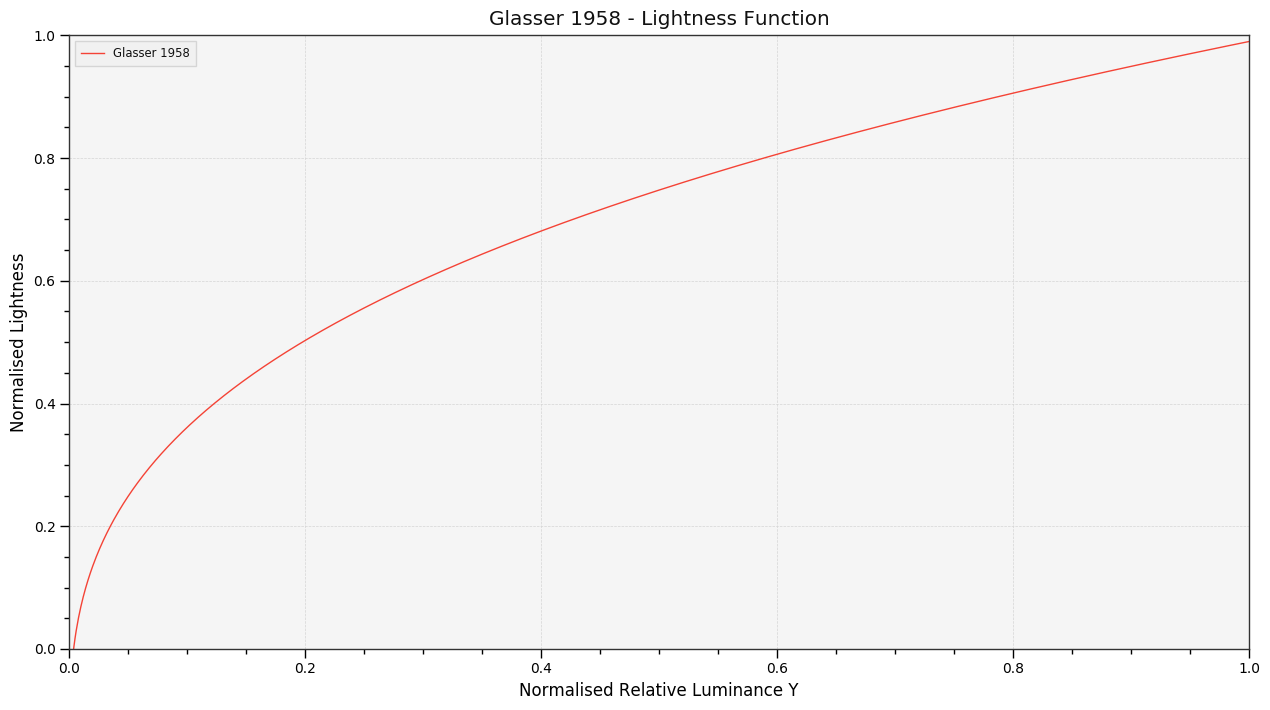## Wyszecki (1963) Method¶

Wyszecki (1963) recommended the following cube root function to compute Lightness $W$ as a function of the luminance factor $Y$ within the practically important range of $1.0\%<Y<98\%$: 

$$\begin{equation} W=25Y^{1/3}-17 \end{equation}$$

The colour.lightness_Wyszecki1963 definition is used to compute Lightness $W$:

In :
colour.colorimetry.lightness_Wyszecki1963(10.08)

Out:
37.004114912764535

Note: Input luminance $Y$ is in domain [0, 100], output Lightness $W$ is in domain [0, 100].

Using the colour.lightness wrapper definition:

In :
colour.lightness(10.08, method='Wyszecki 1963')

Out:
37.004114912764535
In :
# Plotting the "Wyszecki (1963)" "Lightness" function.
with colour.utilities.suppress_warnings():
plot_single_lightness_function('Wyszecki 1963');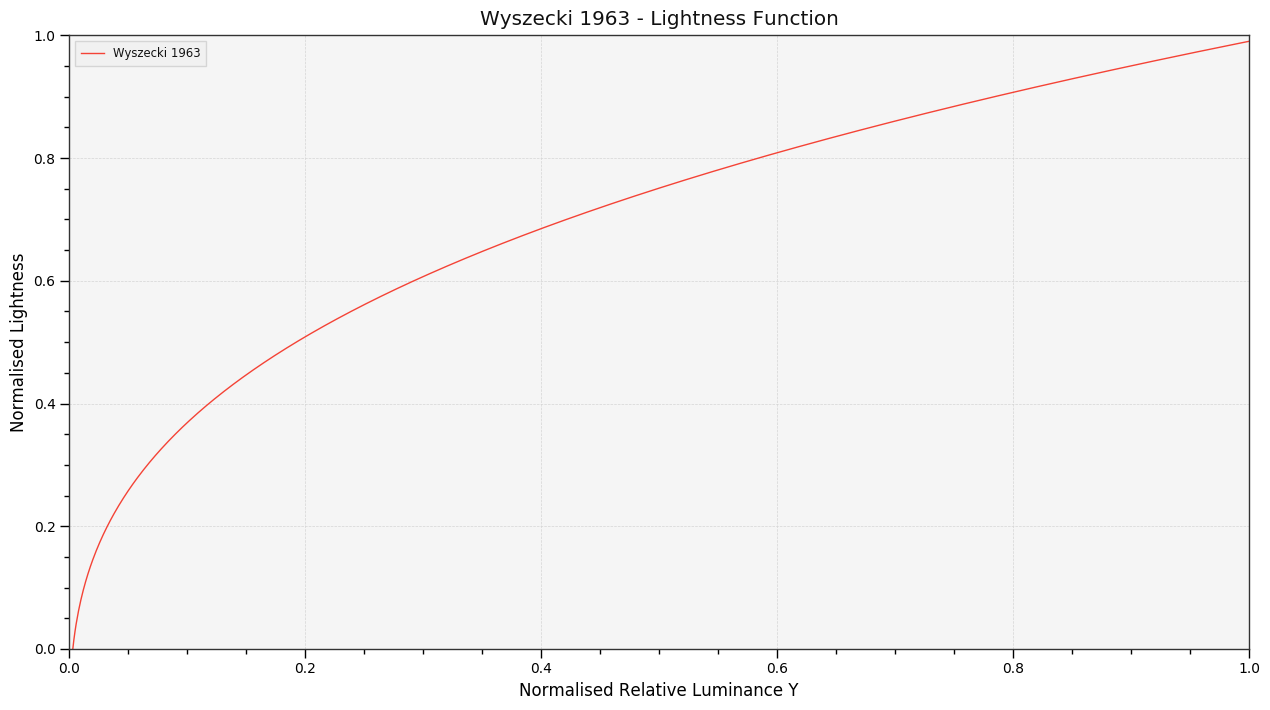## CIE 1976 Method¶

The CIE $L^a^b^$* approximately uniform colourspace defined in 1976 computes the *Lightness* $L^*$ quantity as follows: 

$$\begin{equation} L^*=\begin{cases}116\biggl(\cfrac{Y}{Y_n}\biggr)^{1/3}-16 & for\ \cfrac{Y}{Y_n}>\epsilon\\ \kappa*\biggl(\cfrac{Y}{Y_n}\biggr) & for\ \cfrac{Y}{Y_n}<=\epsilon \end{cases} \end{equation}$$

where $Y_n$ is the reference white luminance. with \begin{equation} \begin{aligned} \epsilon&\ =\begin{cases}0.008856 & Actual\ CIE\ Standard\\ 216\ /\ 24389 & Intent\ of\ the\ CIE\ Standard \end{cases}\\ \kappa&\ =\begin{cases}903.3 & Actual\ CIE\ Standard\\ 24389\ /\ 27 & Intent\ of\ the\ CIE\ Standard \end{cases} \end{aligned} \end{equation}

The original $\epsilon$ and $\kappa$ constants values have been shown to exhibit discontinuity at the junction point of the two functions grafted together to create the Lightness $L^*$ function. 

Colour uses the rational values instead of the decimal values for these constants.

See Also: The CIE $L^*a^*b^*$ Colourspace notebook for in-depth informations about the CIE $L^a^b^$* colourspace.

The colour.lightness_CIE1976 definition is used to compute Lightness $L^*$:

In :
colour.colorimetry.lightness_CIE1976(10.08)

Out:
37.985629097653039

Note: Input luminance $Y$ and $Y_n$ are in domain [0, 100], output Lightness $L^*$ is in domain [0, 100].

Using the colour.lightness wrapper definition:

In :
colour.lightness(10.08)

Out:
37.985629097653039
In :
colour.lightness(10.08, method='CIE 1976', Y_n=95)

Out:
38.916598757092821
In :
colour.lightness(10.08, method='Lstar1976', Y_n=95)

Out:
38.916598757092821
In :
# Plotting the "CIE 1976" "Lightness" function.
plot_single_lightness_function('CIE 1976');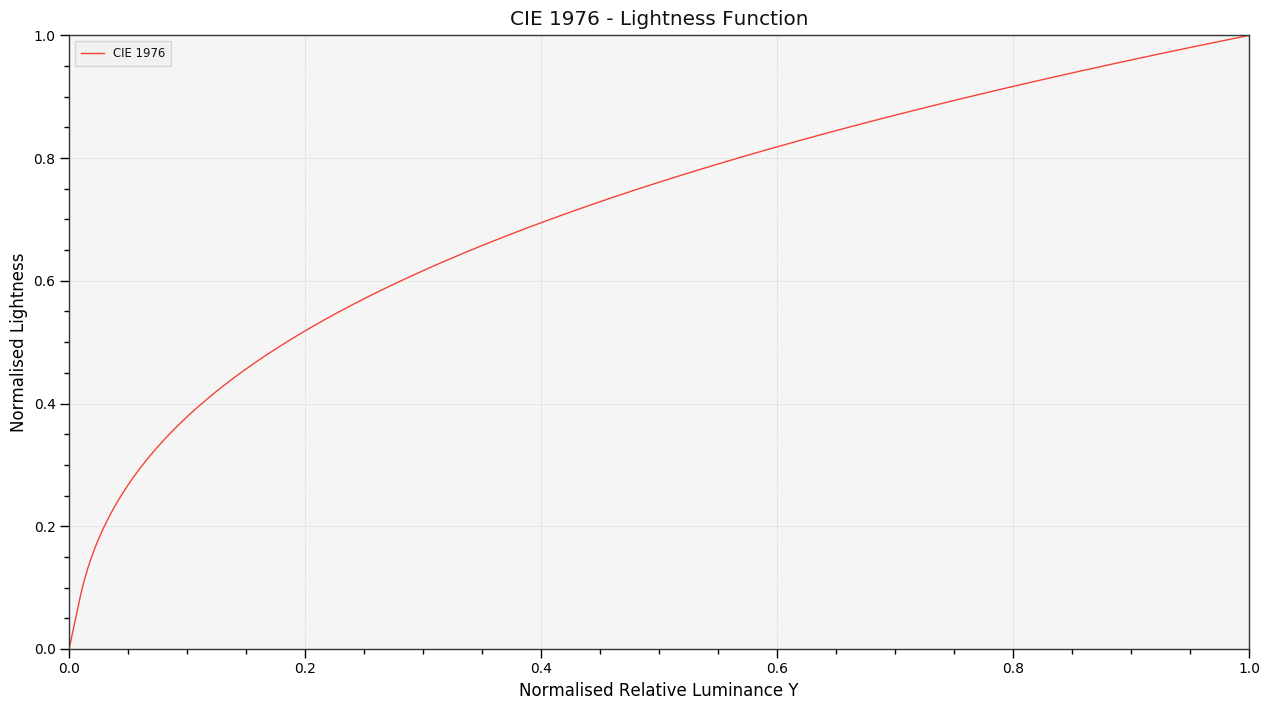In :
# Plotting multiple "Lightness" functions for comparison.
plot_multi_lightness_functions(['CIE 1976', 'Glasser 1958']);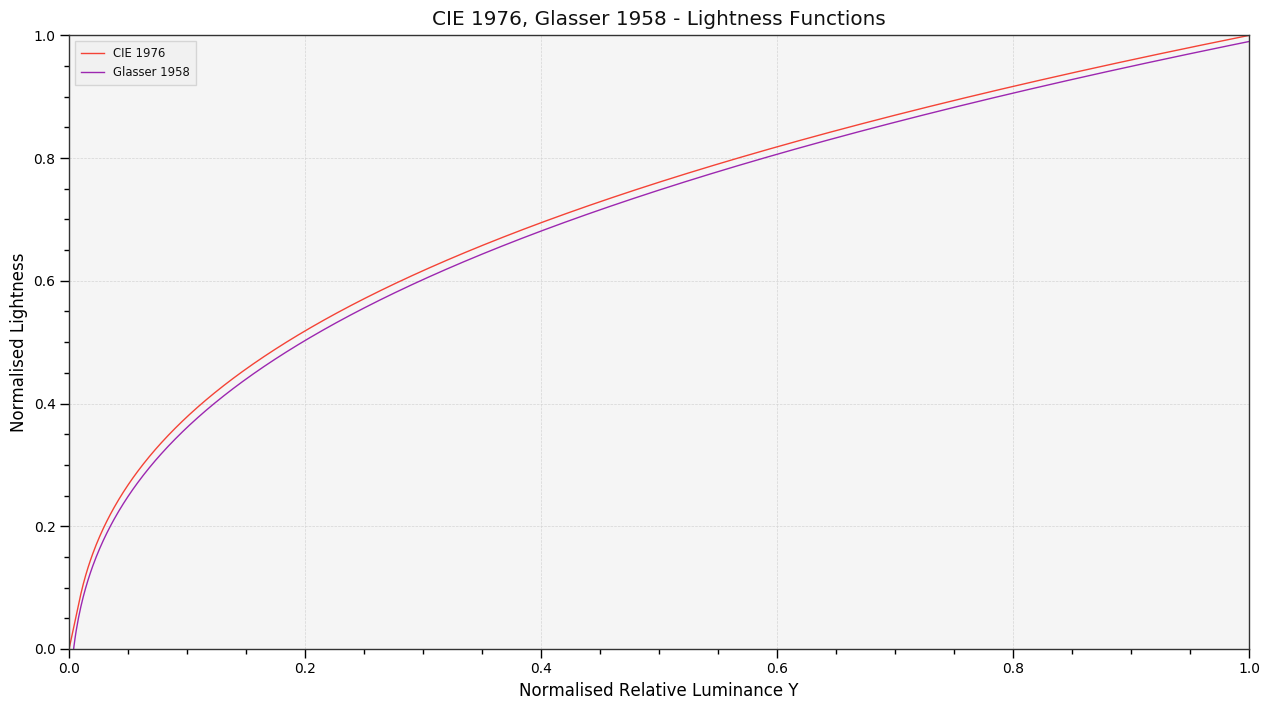## Fairchild and Wyble (2010) Method¶

In :
colour.colorimetry.lightness_Fairchild2010(10.08 / 100, 1.836)

Out:
24.902290269546651
In :
colour.lightness(10.08 / 100, method='Fairchild 2010', epsilon=1.836)

Out:
0.027048835900068357
In :
# Plotting the "Fairchild and Wyble (2010)" "Lightness" function.
plot_single_lightness_function('Fairchild 2010');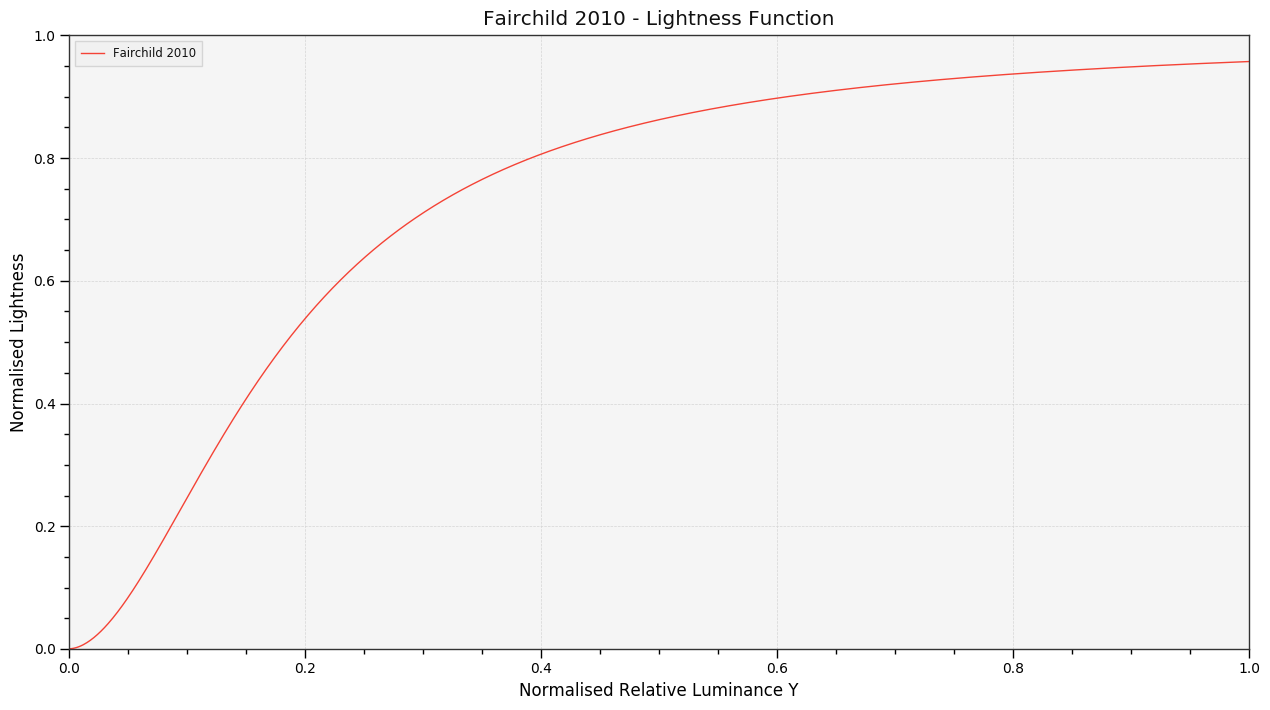In :
# Plotting multiple "Lightness" functions for comparison.
plot_multi_lightness_functions(['CIE 1976', 'Fairchild 2010']);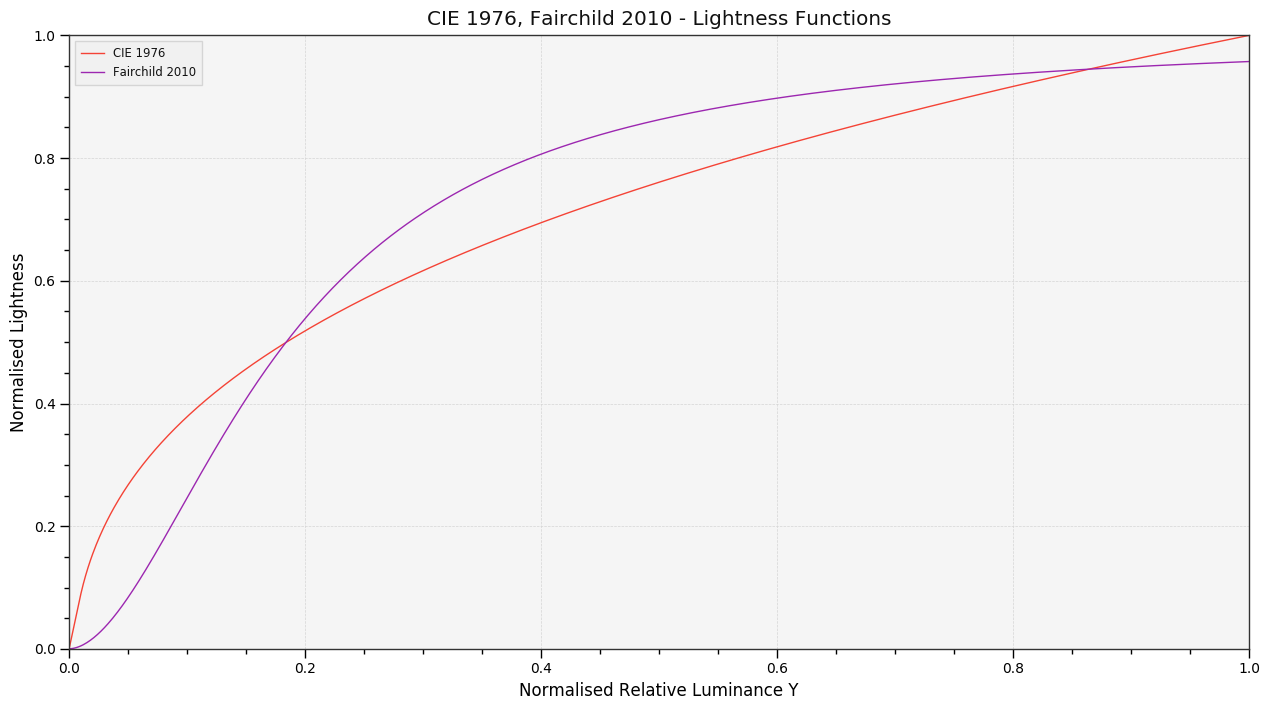## Fairchild and Chen (2011) Method¶

In :
colour.colorimetry.lightness_Fairchild2011(10.08 / 100, 0.710)

Out:
26.459509817572265
In :
colour.lightness(10.08 / 100, method='Fairchild 2011', epsilon=0.710)

Out:
1.1405928270854113
In :
# Plotting the "Fairchild and Chen (2011)" "Lightness" function.
plot_single_lightness_function('Fairchild 2011');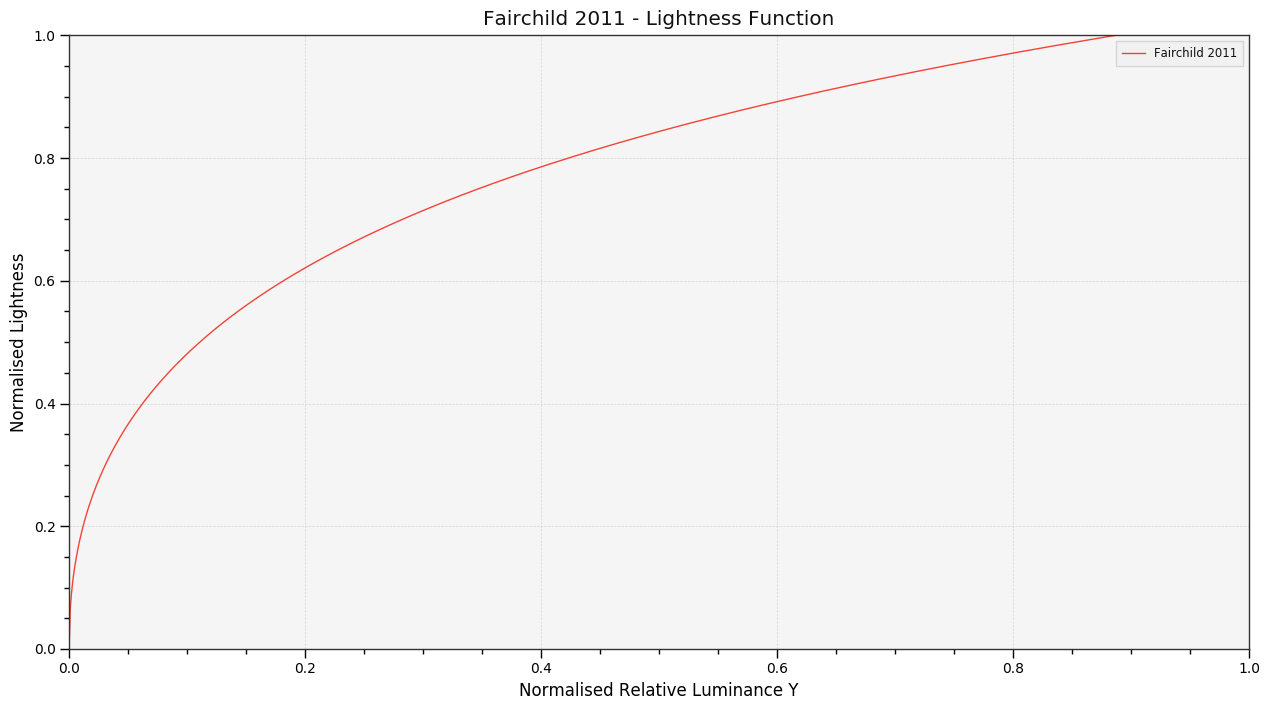In :
# Plotting multiple "Lightness" functions for comparison.
plot_multi_lightness_functions(['CIE 1976', 'Fairchild 2011']);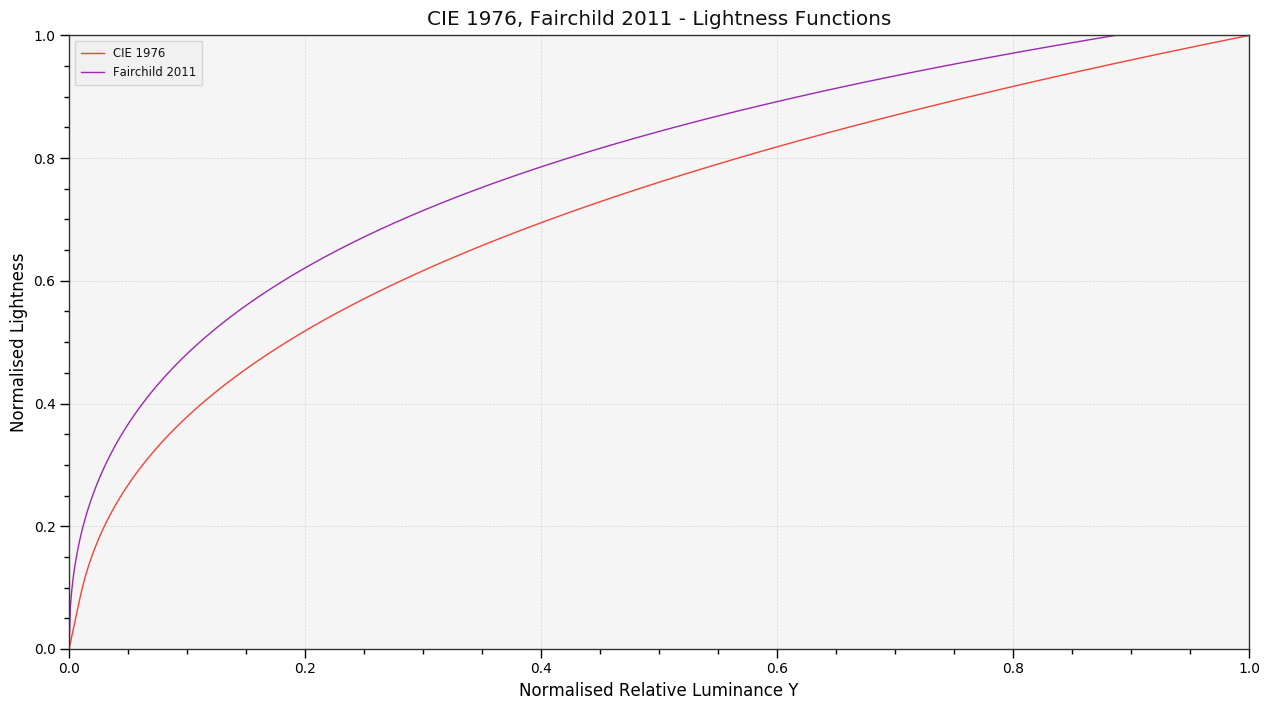## Bibliography¶

1. ^ CIE. (n.d.). 117-680 lightness (of a related colour). Retrieved July 09, 2014, from http://eilv.cie.co.at/term/680
2. ^ Glasser, L. G., McKinney, A. H., Reilly, C. D., & Schnelle, P. D. (1958). Cube-Root Color Coordinate System. J. Opt. Soc. Am., 48(10), 736–740. doi:10.1364/JOSA.48.000736
3. ^ Wyszecki, G. (1963). Proposal for a New Color-Difference Formula. J. Opt. Soc. Am., 53(11), 1318–1319. doi:10.1364/JOSA.53.001318
4. ^ Wyszecki, G., & Stiles, W. S. (2000). CIE 1976 (L*u*v*)-Space and Color-Difference Formula. In Color Science: Concepts and Methods, Quantitative Data and Formulae (p. 167). Wiley. ISBN:978-0471399186
5. ^ Lindbloom, B. (2003). A Continuity Study of the CIE L* Function. Retrieved February 24, 2014, from http://brucelindbloom.com/LContinuity.html